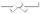# Search Results

Searching for: 2114

## Individual Exercises

0 / 10

Consider the following class definition:

``class Link{ public Object data; public Link next; } ``

In the method below, you will receive a...0 / 50

Write the member method 'playBagBingo()' that implements the player logic for the game described below. Bag Bingo is a game of chance...0 / 50

Write an `ArrayBasedStack<T>` member method called `reverseString()` that takes a String as a parameter, then uses an ArrayBasedStack...0 / 50

Write the toString member method that returns the String representation of the items in the bag in the form [item, item, item, item]. For...0 / 50

Write the `validateMarkup()` standalone method to simulate the behavior of real-world tools used to confirm the validity of web pages.

HTML...0 / 50

Write the `toString()` member method for an ArrayBasedStack. When invoked the method must return the `String` representation of each entry...0 / 50

For this question assume the following code:

`` public class LinkedBag<T> implements BagInterface<T>{ private Node firstNode; ...``0 / 50

For this question assume the following code:

`` public class LinkedBag<T> implements BagInterface<T>{ private Node firstNode; ...``0 / 50

For this question assume the following code:

`` public class LinkedBag<T> implements BagInterface<T>{ private Node firstNode; ...``0 / 50

For this question assume the following code:

`` public class LinkedBag<T> implements BagInterface<T>{ private Node firstNode; ...``0 / 50

delete this question0 / 50

For this question assume the following code:

`` public class LinkedBag<T> implements BagInterface<T>{ private Node firstNode; ...``0 / 50

question text goes here0 / 50

For the question below, assume the following implementation of LinkedStack:

``public class LinkedStack<T> implements StackInterface...``0 / 50

For the question below, assume the following implementation of LinkedStack:

``public class LinkedStack<T> implements StackInterface...``0 / 50

For the question below, assume the following implementation of LinkedStack:

``public class LinkedStack<T> implements StackInterface...``0 / 50

For the question below, assume the following implementation of LinkedQueue:

`` public static final class LinkedQueue<T> implements ...``0 / 50

For the question below, assume the following implementation of LinkedQueue:

`` public static final class LinkedQueue<T> implements ...``0 / 50

For the question below, assume the following implementation of LinkedQueue:

`` public static final class LinkedQueue<T> implements ...``0 / 50

For the question below, assume the following implementation of LinkedQueue:

`` public static final class LinkedQueue<T> implements ...``0 / 50

For the question below, assume the following implementation of ArrayQueue with a fixed-size array and one unused slot

`` public class ...``0 / 50

For the question below, assume the following implementation of ArrayQueue with a fixed-size array and one unused slot

`` public class ...``0 / 50

or the question below, assume the following implementation of LinkedQueue:

`` public class ArrayQueue<T> implements QueueInterface...``0 / 50

For the question below, assume the following implementation of ArrayQueue:

`` public class ArrayQueue<T> implements QueueInterface...``0 / 50

For the question below, assume the following implementation of ArrayQueue:

`` public class ArrayQueue<T> implements QueueInterface...``